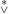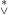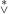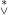#### Vol. 16, No. 3, 1966

 Download this articleFor screen For printingRecent Issues Vol. 307: 1  2 Vol. 306: 1  2 Vol. 305: 1  2 Vol. 304: 1  2 Vol. 303: 1  2 Vol. 302: 1  2 Vol. 301: 1  2 Vol. 300: 1  2Online Archive Volume: Issue:The Journal Officers Subscriptions Editorial Board Submission Guidelines Submission Form Contacts ISSN: 1945-5844 (e-only) ISSN: 0030-8730 (print) Special Issues Author Index To Appear Other MSP Journals
Two notes on regressive isols

### Joseph Barback

Vol. 16 (1966), No. 3, 407–420
##### Abstract

This paper deals with regressive functions and regressive isols. It was proven by J. C. E. Dekker in  that the collection ΛR of all regressive isols is not closed under addition. In the first note of this paper we shall given another proof of this fact by considering a new relation, denoted by, between infinite regressive isols. Let A and B denote infinite regressive isols. The main results established in the first note are:

(1) A BAB, yet not conversely.

(2) A + B ΛRAB, yet not conversely.

(3) There exist infinite regressive isols which are notrelated.

(4) ΛR is not closed under addition.

In addition, the following result is stated.

(5) A + B ΛRmin(A,B) A + B, yet not conversely.

In the second note we consider the relation between regressive isols. A natural question concerning this relation is whether A B, where A and B are regressive isols, is a necessary or a sufficient condition for the sum A + B to be regressive. In the second note we show that this condition is neither necessary nor sufficient.

We shall assume that the reader is familiar with the notations, terminology and main results of  and .

Primary: 02.70
##### Milestones